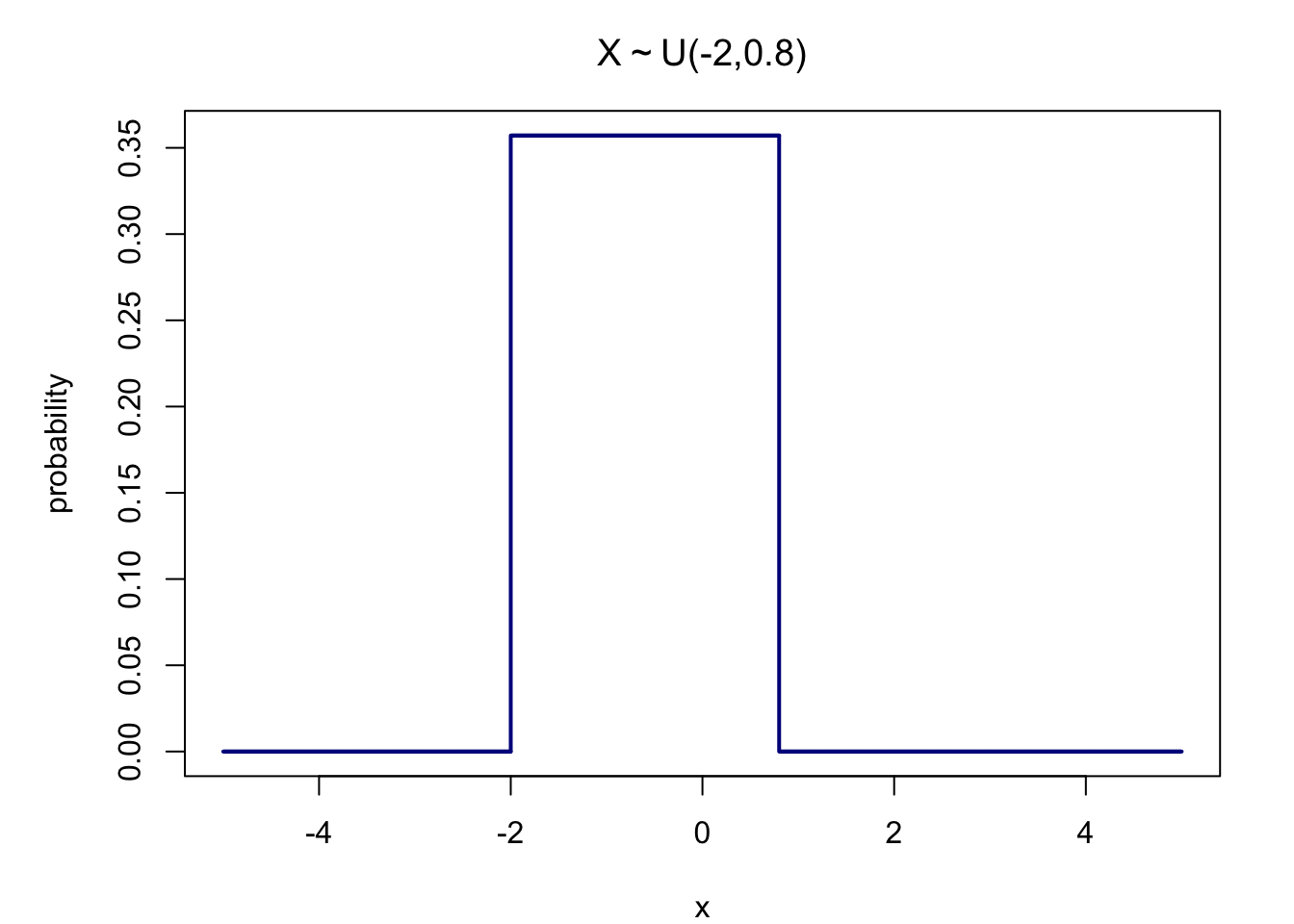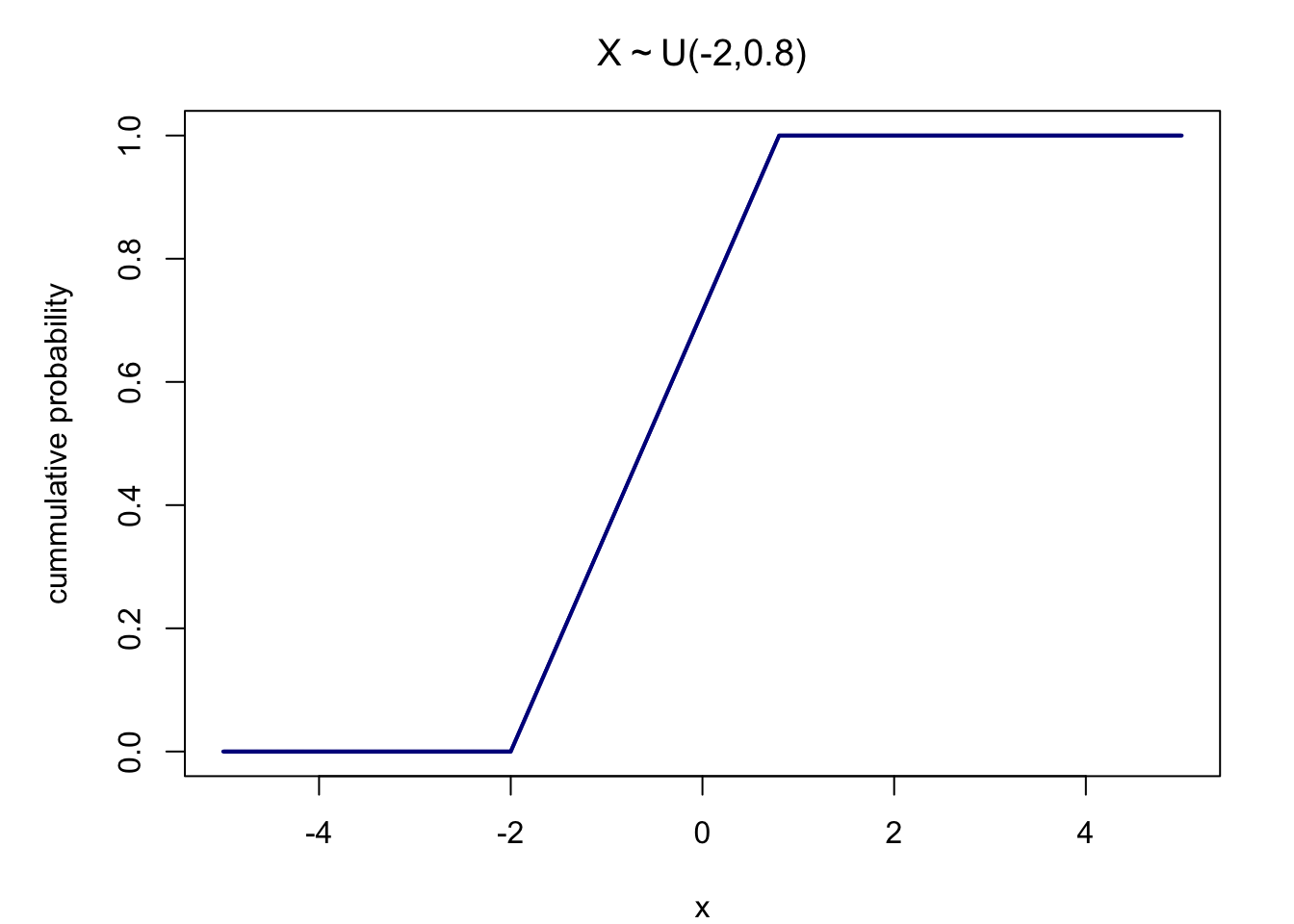The Uniform distribution is the simplest probability distribution, but it plays an important role in statistics since it is very useful in modeling random variables. The uniform distribution is a continuous probability distribution and is concerned with events that are equally likely to occur. The continuous random variable $$X$$ is said to be uniformly distributed, or having rectangular distribution on the interval $$[a, b]$$. We write $$X \sim U(a, b)$$, if its probability density function equals $$f(x) = \frac{1}{b-a}, \; x \in [a,b]$$ and $$0$$ elsewhere (Lovric 2011).

$f(x) = \begin{cases} \frac{1}{b-a}, & \text{when a \le x \le b} \\[2ex] 0, & \text{when x < a or x > b} \end{cases}$

The figure below shows a continuous uniform distribution $$X \sim U(-2, 0.8)$$, thus a distribution where all values of $$x$$ within the interval [-2,0.8] are $$\frac{1}{b-a} (=\frac{1}{0.8-(-2)} = 0.36)$$, whereas all other values of $$x$$ are 0.The mean and the median are given by $\mu = \frac{a+b}{2}\text{.}$

The cumulative density function is shown below and given be the equation

$F(x) = \begin{cases} 0, & \text{for x < a} \\[2ex] \frac{x-a}{b-a}, & \text{for x \in [a,b)} \\[2ex] 1, & \text{for x \ge b} \end{cases}$